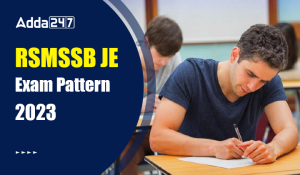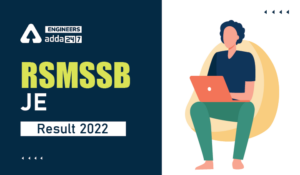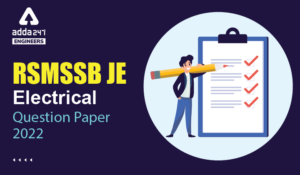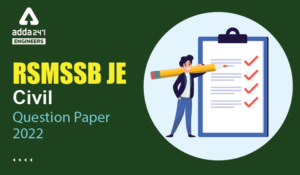Engineering Jobs   »   Civil Engineering Quiz

# RSMSSB-JE’21 CE: Weekly Practices Quiz. 22-Aug-2021

Quiz: Civil Engineering
Exam: RSMSSB-JEn
Topic: Miscellaneous

Each question carries 1 mark
Negative marking: 1/4 mark
Time: 12 Minutes

Q1. The process of providing smooth face and regular face to stone is known as
(a) Seasoning
(b) Dressing
(c) Pitching
(d) Quarrying

Q2. In limit state method of design, for bars in compression the values of bond stress shall be:
(a) Decreased by 20%
(b) Increased by 25%
(c) Decreased by 25%
(d) Increased by 20%

Q3. Which of the following related fluid flow parameter exist both in rotational and irrotational flows?
(a) Vorticity
(b) Stream function
(c) Velocity potential
(d) Both vorticity and stream function

Q4. Creep is the gradual increase of
(a) plastic strain with time at constant load
(b) elastic strain with time at constant load
(c) plastic strain with time at varying load
(d) elastic strain with time at varying load

Q5. Which of the following is the correct sequence of plasticity of minerals in soil in an increasing order?
(a) Kaolinite, silica, montmorillonite, illite
(b) Kaolinite, silica, illite, montmorillonite
(c) Silica, kaolinite, illite, montmorillonite
(d) Silica, kaolinite, montmorillonite, illite

Q6. What is the mechanical widening required for a pavement of width 7m on a horizontal curve of radius 490m, if the longest wheel base of vehicle expected on the road is 7.0m?
(a) 0.07 m
(b) 0.05 m
(c) 0.49m
(d) 0.1m

Q7. In case of a direct vernier scale
(a) graduations increase in opposite direction in which graduations of the main scale increase
(b) smallest division is longer than smallest division of the main scale
(c) graduations increase in the same direction in which graduations of the main scale increase
(d) None of these

Q8. What is the presence of original rounded surface on the manufactured piece of timber called?
(a) Chipmark
(b) Torn grain
(c) Wane
(d) Diagonal grain

Q9. Aerobic attached growth process is classified under:
(a) activated sludge process
(b) chemical clarification
(c) biological treatment
(d) preliminary treatment

Q10. The relationship, between number of joints (j), and the number of members (m), in a perfect truss, is given by
(a) m = 3j – 2
(b) m = 2j – 3
(c) m = j – 2
(d) m = 2j – 1

Q11. The term ‘Characteristic load’ means that load which has a probability of not being exceeded, during the life of the structure is equal to:
(a) 90%
(b) 95%
(c) 99%
(d) 100%

Q12. If angle of internal friction of soil is 30°, coefficient of active earth pressure will be:
(a) 1/2
(b) 1/3
(c) 1/4
(d) 2/3

Solutions

S1. Ans.(b)
Sol. Dressing → Stone dressing is the process of providing proper shape and size to fresh quarried stone.
OR
It is the process in which surface of stone is made fit to be used in any construction purpose.

S2. Ans.(b)
Sol. Table of bond stress in IS code given for plain bars in tension.
→ for HYSD bar in tension increase the value by 60%
→ for bars in compression the value is increased by 25%

S3. Ans.(b)
Sol. Vorticity is defined only for rotational flow. Stream function is defined for both rotational and irrotational flow. The velocity potential function exists for only irrotational flow.

S4. Ans.(a)
Sol. Creep is the property of a specimen undergoes additional deformation with the passage of time under sustained loading within elastic limit. It cannot be recovered hence plastic in nature.

S5. Ans.(c)
Sol. Increasing order of plasticity in clay mineral –
Silica → kaolinite → Illite → montmorillonite

S6. Ans.(d)
Sol. Given, length of wheel base (l) = 7m
No. of lanes (n) = 2
mechanical widening W_me = (nl^2)/2R
= (2×(7)^2)/(2×490) = 0.1

S7. Ans.(c)
Sol. Direct vernier – division which are slightly shorter than main scale. Reading increases in the direction of the main scale.
→ n division of vernier is equal to (n-1) division of main scale.
Retrograde vernier – Division which are slightly longer than main scale. Reading increases in a direction opposite to main scale. n division of vernier (n+1) division of main scale.

S8. Ans.(c)
Sol. Wane → Presence of original rounded surface on the manufactured piece of timber called wane.
Torn grain→ it is the depression induced in timber due to falling of tools over timber.
Chip mark → This is the detect in timber indicated by mark or sign placed on finished surface of timber.

S9. Ans.(c)
Sol. Aerobic attached growth process is a biological treatment in which biomass is attached over the medium and waste water passed through it.

S10. Ans.(b)
Sol. The relationship, between number of joints (j), and the number of members (m), in a perfect truss, is given by –
m = 2j-3
Where m = No. of truss member
J =No. of joint in the truss.

S11. Ans.(b)
Sol. Characteristic load means that load which has 95% probability of not being Exceeded during entire life of structure.

S12. Ans.(b)
Sol. Angle of internal frictions (ϕ) =30 coefficient of Active earth pressure (K_a)=
1-sinϕ
=(1-sinϕ)/(1+sinϕ)
=(1-sin30)/(1+sin30)
=1/3

Sharing is caring!

### TOPICS:

•RSMSSB JE Exam Pattern 2023, Check Lates...
•RSMSSB JE 2022 DV Schedule, Download RSM...
•RSMSSB JE Result 2022, OUT-Direct Link t...
•RSMSSB Computer Instructor Exam date 202...
•RSMSSB JE Electrical Question Paper 2022...
•RSMSSB JE Civil Question Paper 2022, Che...